##### Algebra II For Dummies, 2nd EditionSome algebraic equations that contain radicals call for more than one application of squaring both sides. For example, you usually need to square both sides more than once when you have three terms in the equation — two of them with radicals.

For example, say you have to work with the equation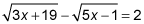Here's how you solve the problem:

1. Move the radicals so that only one appears on each side.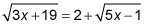2. Square both sides of the equation.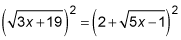After simplifying the results of the first two steps, you have the following: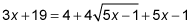3. Move all the nonradical terms to the left and simplify.

This gives you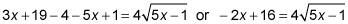4. Make the job of squaring the binomial on the left easier by dividing each term by two — the common factor of all the terms on both sides.

You end up with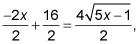which becomes5. Square both sides again, simplify, set the quadratic equal to zero, and solve for x.

This process gives you the following: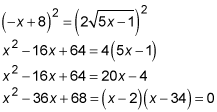When x – 2 = 0, x = 2; and when x – 34 = 0, x = 34.

6. Don't forget to check each solution in the original equation: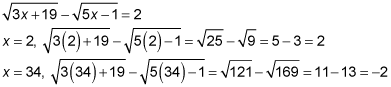The solution x = 2 works. The other solution, x = 34, doesn't work in the equation. The number 34 is an extraneous solution.